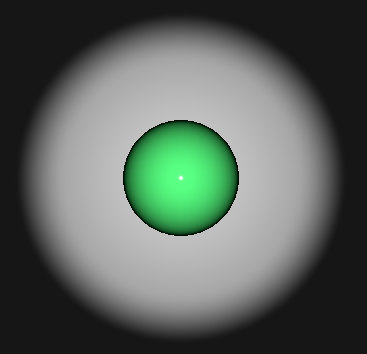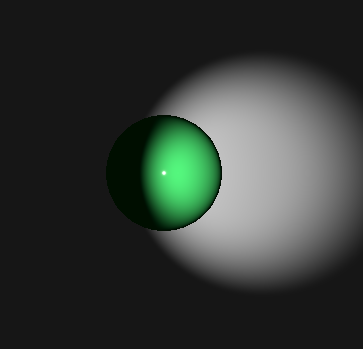# SpotLight QML Type

Defines a spot light in the scene. More...

 Import Statement: import QtQuick3D Since: Qt 5.15 Inherits: Light

## Detailed Description

The spot light emits light towards one direction in a cone shape, which is defined by the coneAngle property. The light intensity diminishes when approaching the coneAngle. The angle at which the light intensity starts to diminish is defined by innerConeAngle. Both angles are defined in degrees.

Inside the innerConeAngle, the spot light behaves similarly to the point light. There the light intensity diminishes according to inverse-square-law. However, the fade-off (and range) can be controlled with the constantFade, linearFade, and quadraticFade properties. Light attenuation is calculated using the formula: constantFade + `distance` * (linearFade * 0.01) + `distance` * (quadraticFade * 0.0001)^2

Let's look at a simple example. Here a SpotLight is placed at 300 on the Z axis, so halfway between the camera and the scene center. By default the light is emitting in the direction of the Z axis. The brightness is increased to 10 to make it look more like a typical spot light.

```import QtQuick
import QtQuick3D
View3D {
anchors.fill: parent

PerspectiveCamera { z: 600 }

SpotLight {
z: 300
brightness: 10
ambientColor: Qt.rgba(0.1, 0.1, 0.1, 1.0)
}

Model {
source: "#Rectangle"
scale: Qt.vector3d(10, 10, 10)
z: -100
materials: PrincipledMaterial { }
}

Model {
source: "#Sphere"
scale: Qt.vector3d(2, 2, 2)
materials: PrincipledMaterial {
baseColor: "#40c060"
roughness: 0.1
}
}
}```Rotations happens similarly to DirectionalLight. Here we want to light to emit more to the right, so we rotate around the Y axis by -20 degrees. The cone is reduced by setting coneAngle to 30 instead of the default 40. We also make the intensity start diminish earlier, by changing innerConeAngle to 10.

```SpotLight {
z: 300
brightness: 10
ambientColor: Qt.rgba(0.1, 0.1, 0.1, 1.0)
eulerRotation.y: -20
coneAngle: 30
innerConeAngle: 10
}```For further usage examples, see Qt Quick 3D - Lights Example.

## Property Documentation

 coneAngle : real

This property defines the cut-off angle (from edge to edge) beyond which the light doesn't affect the scene. Defined in degrees between 0 and 180. The default value is 40.

This property is constant factor of the attenuation term of the light. The default value is 1.0.

 innerConeAngle : real

This property defines the angle (from edge to edge) at which the light intensity starts to gradually diminish as it approaches coneAngle. Defined in degrees between 0 and 180. If the value is set larger than coneAngle, it'll behave as if it had the same value as coneAngle. The default value is 30.

This property increases the rate at which the lighting effect dims the light in proportion to the distance to the light. The default value is `0.0`, which means the light doesn't have linear fade. The value used here is multiplied by `0.01` before being used to calculate light attenuation.
This property increases the rate at which the lighting effect dims the light in proportion to the inverse square law. The default value is 1.0, which means the spot light fade exactly follows the inverse square law, i.e. when distance to an object doubles the light intensity decreases to 1/4th. The value used here is multiplied by `0.0001` before being used to calculate light attenuation.